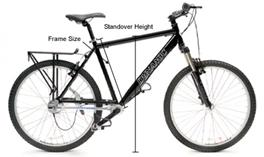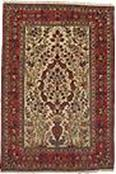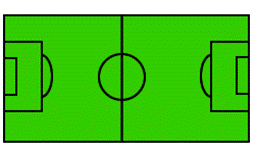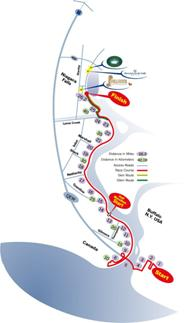Units of Length

LEARNING outcomes

• Define units of length and convert from one to another
• Perform arithmetic calculations on units of length
• Solve application problems involving units of length

Suppose you want to purchase tubing for a project, and you see two signs in a hardware store: $1.88 for 2 feet of tubing and$5.49 for 3 yards of tubing. If both types of tubing will work equally well for your project, which is the better price? You need to know about two units of measurement, yards and feet, in order to determine the answer.

Length is the distance from one end of an object to the other end, or from one object to another. For example, the length of a letter-sized piece of paper is 11 inches. The system for measuring length in the United States is based on the four customary units of length: inch, foot, yard, and mile. Below are examples to show measurement in each of these units.

 Unit Description Image Inch/Inches Some people donate their hair to be made into wigs for cancer patients who have lost hair as a result of treatment. One company requires hair donations to be at least 8 inches long.Frame size of a bike: the distance from the center of the crank to the top of the seat tube. Frame size is usually measured in inches. This frame is 16 inches.Foot/Feet Rugs are typically sold in standard lengths. One typical size is a rug that is 8 feet wide and 11 feet long. This is often described as an 8 by 11 rug.Yard/Yards Soccer fields vary some in their size. An official field can be any length between 100 and 130 yards.Mile/Miles A marathon is 26.2 miles long. One marathon route is shown in the map to the right.You can use any of these four U.S. customary measurement units to describe the length of something, but it makes more sense to use certain units for certain purposes. For example, it makes more sense to describe the length of a rug in feet rather than miles, and to describe a marathon in miles rather than inches.

You may need to convert between units of measurement. For example, you might want to express your height using feet and inches (5 feet 4 inches) or using only inches (64 inches). You need to know the unit equivalents in order to make these conversions between units.

The table below shows equivalents and conversion factors for the four customary units of measurement of length.

 Unit Equivalents Conversion Factors (longer to shorter units of measurement) Conversion Factors(shorter to longer units of measurement) 1 foot = 12 inches $\displaystyle \frac{12\ \text{inches}}{1\ \text{foot}}$ $\displaystyle \frac{1\text{ foot}}{12\text{ inches}}$ 1 yard = 3 feet $\displaystyle \frac{3\text{ feet}}{1\text{ yard}}$ $\displaystyle \frac{\text{1 yard}}{\text{3 feet}}$ 1 mile = 5,280 feet $\displaystyle \frac{5,280\text{ feet}}{1\text{ mile}}$ $\displaystyle \frac{\text{1 mile}}{\text{5,280 feet}}$

Note that each of these conversion factors is a ratio of equal values, so each conversion factor equals 1. Multiplying a measurement by a conversion factor does not change the size of the measurement at all since it is the same as multiplying by 1; it just changes the units that you are using to measure.

recall simplifying fractions

Conversion factors must contain the same number in the top and in the bottom in order to equal 1. Since these are ratios of equal values, they are also fractions. And since a fraction is a division problem, we can apply the same principle we use to simplify fractions,

$\dfrac{a}{a}=1$.

Convert Between Different Units of Length

You can use the conversion factors to convert a measurement, such as feet, to another type of measurement, such as inches.

Note that there are many more inches for a measurement than there are feet for the same measurement, as feet is a longer unit of measurement. You could use the conversion factor $\displaystyle \frac{\text{12 inches}}{\text{1 foot}}$.

If a length is measured in feet, and youd like to convert the length to yards, you can think, I am converting from a shorter unit to a longer one, so the length in yards will be less than the length in feet. You could use the conversion factor $\displaystyle \frac{\text{1 yard}}{\text{3 feet}}$.

If a distance is measured in miles, and you want to know how many feet it is, you can think, I am converting from a longer unit of measurement to a shorter one, so the number of feet would be greater than the number of miles. You could use the conversion factor $\displaystyle \frac{5,280\text{ feet}}{1\text{ mile}}$.

You can use the factor label method (also known as dimensional analysis) to convert a length from one unit of measure to another using the conversion factors. In the factor label method, you multiply by unit fractions to convert a measurement from one unit to another. Study the example below to see how the factor label method can be used to convert $\displaystyle 3\frac{1}{2}$ feet into an equivalent number of inches.

Convert a mixed number to an improper fraction

You can use a handy shortcut to convert a mixed number to its equivalent fractional form. First multiply the whole number part by the denominator of the fraction then add the numerator to the result. Finally place that number over the denominator.

$a\dfrac{b}{c}=\dfrac{ac+b}{c}$.

Ex. Convert $3\dfrac{1}{2}$ to an improper fraction.

$3\dfrac{1}{2}=\dfrac{3\cdot 2 + 1}{2}=\dfrac{7}{2}$.

Example

How many inches are in $\displaystyle 3\frac{1}{2}$ feet?

Notice that by using the factor label method you can cancel the units out of the problem, just as if they were numbers. You can only cancel if the unit being cancelled is in both the numerator and denominator of the fractions you are multiplying.

In the problem above, you cancelled feet and foot leaving you with inches, which is what you were trying to find.

$\frac{7}{2}\cancel{\text{feet}}\cdot\frac{12\text{ inches}}{\cancel{1\text{foot}}}=\text{? inches}$

What if you had used the wrong conversion factor?

$\frac{7}{2}\text{feet}\cdot\frac{1\text{foor}}{12\text{ inches}}=\text{? inches}$?

You could not cancel the feet because the unit is not the same in both the numerator and the denominator. So if you complete the computation, you would still have both feet and inches in the answer and no conversion would take place.

Here is another example of a length conversion using the factor label method.

Convert an improper fraction to a mixed number

To convert a mixed number to an improper fraction, you’ll need to consider how many times the denominator divides into the numerator and if there is a remainder.

Ex. Convert the improper fraction $\dfrac{13}{5}$ to a mixed number.

The fraction bar is a division symbol.$\dfrac{13}{5} = 13 \div 5$.

$13 \div 5 = 2$ with $3$ left over, which we place over the divisor, $5$.

That is, the result of the division is $2$ and $\dfrac{3}{5}$, which we write as the mixed number

$2\dfrac{3}{5}$.

Example

How many yards is $7$ feet?

Apply Unit Conversions With Length

There are times when you will need to perform computations on measurements that are given in different units. For example, consider the tubing problem given earlier. You must decide which of the two options is a better price, and you have to compare prices given in different unit measurements.

In order to compare, you need to convert the measurements into one single, common unit of measurement. To be sure you have made the computation accurately, think about whether the unit you are converting to is smaller or larger than the number you have. Its relative size will tell you whether the number you are trying to find is greater or lesser than the given number.

Example

An interior decorator needs border trim for a home she is wallpapering. She needs 15 feet of border trim for the living room, 30 feet of border trim for the bedroom, and 26 feet of border trim for the dining room. How many yards of border trim does she need?

Try It

The next example uses the factor label method to solve a problem that requires converting from miles to feet.

Example

Two runners were comparing how much they had trained earlier that day. Jo said, “According to my pedometer, I ran 8.3 miles.” Alex said, “That’s a little more than what I ran. I ran 8.1 miles.” How many more feet did Jo run than Alex?

In the next example we show how to compare the price of two different kinds of tubing for a project you are making. One type of tubing is given in cost per yards, and the other is given in cost per feet. It is easier to make a comparison when the units are the same, so we convert one price into the same units as the other. For problems like this, it doesn’t matter which cost you convert, either one will work.

Example

You are walking through a hardware store and notice two sales on tubing:

• 3 yards of Tubing A costs $5.49. • Tubing B sells for$1.88 for 2 feet.

Either tubing is acceptable for your project. Which tubing is less expensive?

In the problem above, you could also have found the price per foot for each kind of tubing and compared the unit prices of each per foot.

You need to convert from one unit of measure to another if you are solving problems that include measurements involving more than one type of measurement. Each of the units can be converted to one of the other units using the table of equivalents, the conversion factors, and/or the factor label method shown in this topic.The four basic units of measurement that are used in the U.S. customary measurement system are: inch, foot, yard, and mile. Typically, people use yards, miles, and sometimes feet to describe long distances. Measurement in inches is common for shorter objects or lengths.